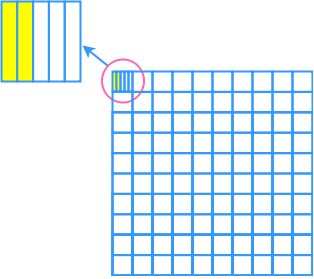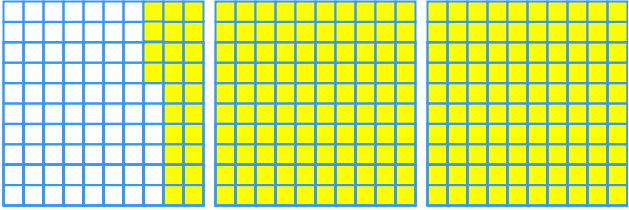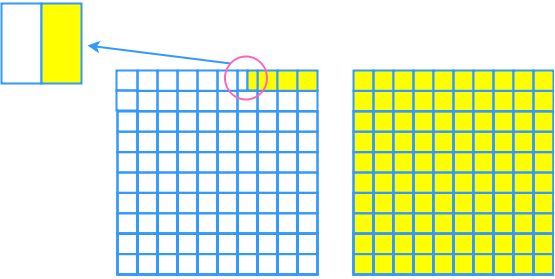# Representing percents

### Representing percents

Percentages can be an abstract concept when you start learning it. In this section, we will use grids and diagrams to help you understand the concept better.

#### Lessons

• Introduction
a)
What is Percent?

• 1.
One fully shaded grid represents 100%. What percent does each of the following diagrams represent?
a)b)c)• 2.
Represent the percent by using grids.
a)
The sales of coffee makes up about 75% of the total profit of a café.

b)
The apple harvest increased by 2.5% this year.

c)
The height of Ken is 130% of the height of Mary.